# Fisseha Berhane, PhD

## Issues to pay attention to when performing PCA in Spark, Python and R¶

Principal component analysis (PCA) is a statistical analysis technique that transforms possibly correlated variables to orthogonal linearly uncorrelated values. We can use it for applications such as data compression, to get dominant physical processes and to get important features for machine learning. Without losing much information, it reduces the number of features in the original data.

Recently, I was using PCA with Spark with sparse matrix with millions of rows and to make sure everything was right I first started with a small dataset and compared the results from Spark, Python and R which led to this blog post.

In this post, I will cover data prepocessing required and how to implement PCA in R, Python and Spark and how to translate the results.

The calculation is done using singular value decomposition (SVD). The SVD of any m x n matrix is calculated as

$A = U \sum V^{T}$

where U is an m × m orthogonal matrix whose columns are the eigenvectors of $AA^{T}$ , V is an n × n orthogonal matrix whose columns are the eigenvectors of $A^{T}A$, and Σ is an m × n diagonal matrix and its values are zero except along the diagonal.

When applying PCA, we have to center our data that is we have to subtract the column mean. Next, based on the nature of our data, we may need to standardize our data (make each feature have a unit variance and zero mean). If the columns are in different scales such as year, temperature, carbondioxide concentration, for example, we have to standardize the data. If the data is in the same unit, on the other hand, standardizing it can result in loss of important information. In the first case, when the columns are in the same unit and in similar scale, we use the covariance matrix for SVD but when the units are different since we standardize the data, we use the correlation matrix.

The principal components (PCs) are the matrix product of the original data and the matrix V which is equal to the product of matrixes U and ∑.

### Variables in the same unit¶

For this example, we will use the iris data. The data set consists of 50 samples from each of three species of Iris (Iris setosa, Iris virginica and Iris versicolor). Each sample has four features: the length and the width of the sepals and petals, in centimeters. You can get the data, including details, from UCI Machine Learning Repository. I downloaded the data from here

In :
options(repr.plot.width=8, repr.plot.height=5)


### R¶

In :
library(tidyverse)

library(corrplot)

In :
iris = read_csv("https://raw.githubusercontent.com/venky14/Machine-Learning-with-Iris-Dataset/master/Iris.csv")

In :
head(iris)    # show sample records

IdSepalLengthCmSepalWidthCmPetalLengthCmPetalWidthCmSpecies
1 5.1 3.5 1.4 0.2 Iris-setosa
2 4.9 3.0 1.4 0.2 Iris-setosa
3 4.7 3.2 1.3 0.2 Iris-setosa
4 4.6 3.1 1.5 0.2 Iris-setosa
5 5.0 3.6 1.4 0.2 Iris-setosa
6 5.4 3.9 1.7 0.4 Iris-setosa

All the units are in cm. Since we do not need the Id and Species columns for our analysis, let's drop them.

In :
species = iris$Species # creating a vector to use it later iris = iris %>% select(-Id,-Species)  Since the columns are in the same scale, we do not need to scale it: just centering the columns is enough (SVD uses covariance matrix in this case). As shown below, we can specify if we want to center it or scale it. In : correlations = cor(iris) corrplot(correlations, method="circle", addCoef.col = "white", title = 'Fig.1. Correlation', mar=c(0,0,1,0))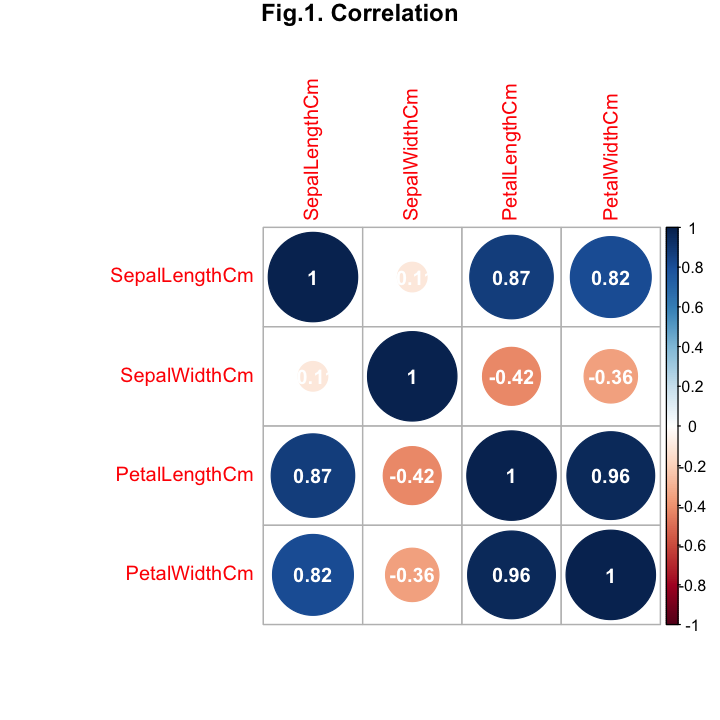As shown in Fig.1. above, some of the variables are strongly correlated. This means we can reduce the dimensionality with out losing information. When the variables are strongly correlated, the first few PCs explain large portion of the variability in the data. When applying PCA with R, Python or Spark, we have to make sure that the rows are samples and the columns are variables. #### Apply PCA¶ In : pcp = prcomp(iris, center = TRUE, scale. = FALSE) # center it but do not scale it  The prcomp function uses SVD and returns the following: 1. x: principal components which are the matrix product of the original data and the rotation: x = df %*% rotation 2. sdev: standard deviation scaled by sample size in an unbiased way (ie. 1/(n-1)) 3. rotation: loadings of each principal component #### Variance Explained¶ The variance explained by each PC is shown below. The PCs are ranked based on the variance they explain: the first PC explains the highest variance, followed by the second PC and so on. So, we see that the first PC explains almost all the variance (92.4616%) while the fourth PC explains only 0.5183%. In such scenarios where we have many columns and the first few PCs account for most of the variability in the original data (such as 95% of the variance), we can use the first few PCs for data explorations and for machine learning. In data explorations, we can visualize the PCs and get a better understanding of processes using those PCs. In machine learning, on the other hand, by using the PCs, we will work with less number of columns and this can significantly reduce computational cost and help in reducing over-fitting. It is important to mention here that when you use PCA for machine learning, the test data should be transformed using the loading vectors found from the training data otherwise your model will have difficulty generalizing to unseen data. In : variance = data_frame(PC = paste0('PC', seq(1,4)), variance = round(100.00*pcp$sdev^2/sum(pcp$sdev^2),4)) variance %>% ggplot(aes(x = PC, y = variance)) + geom_bar(stat = 'identity') + ggtitle('Fig.2. Variance Explained') + theme(axis.title = element_text(size=14), plot.title = element_text(size = 16,colour="darkblue", hjust = 0.5), axis.text = element_text(size=14)) + geom_text(aes(label = variance), vjust = -0.4) + xlab('') + ylab('Variance Explained (%)')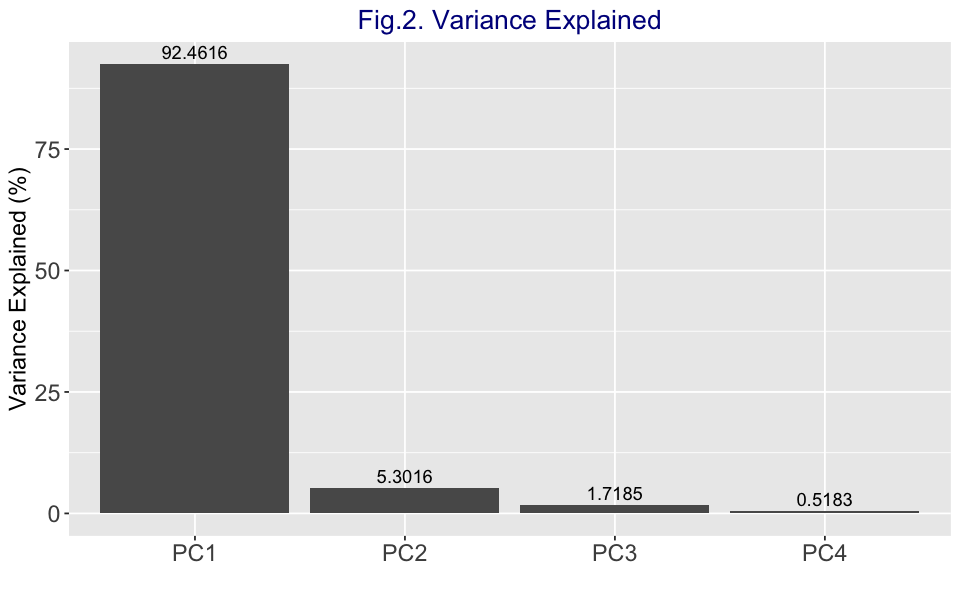#### Loadings¶ We can see which variables have the largest effects in each PC from the rotations (loadings). The features which have the highest magnitude of loadings in the first PC have the highest effect. From the loading of the iris data below, we observe that petal length has the highest effect in the first PC while in the second PC sepal length and sepal width are the most important. If two features have similar loadings in the first and the second PCs, the one in the first PC is more important. Generally, for similar loadings, features in the$n^{th} -1$PC are more important than those in the$n^{th}$PC. In : round(pcp$rotation, 4)

PC1PC2PC3PC4
SepalLengthCm 0.3616-0.6565 0.5810 0.3173
SepalWidthCm-0.0823-0.7297-0.5964-0.3241
PetalLengthCm 0.8566 0.1758-0.0725-0.4797
PetalWidthCm 0.3588 0.0747-0.5491 0.7511
In :
rotation = as.data.frame(pcp$rotation) rotation$dimension = row.names(rotation)
rotation = rotation %>% melt(key = dimension, value = pc) %>% mutate(magnitude = abs(value))
rotation %>% ggplot(aes(x  = dimension, y = variable , fill = magnitude)) + geom_tile()+xlab('') + ylab('') +
theme(axis.title = element_text(size=14), plot.title = element_text(size = 18,colour="darkblue", hjust = 0.5),
axis.text = element_text(size=14)) + geom_text(aes(label = round(magnitude,3)))

Using dimension as id variables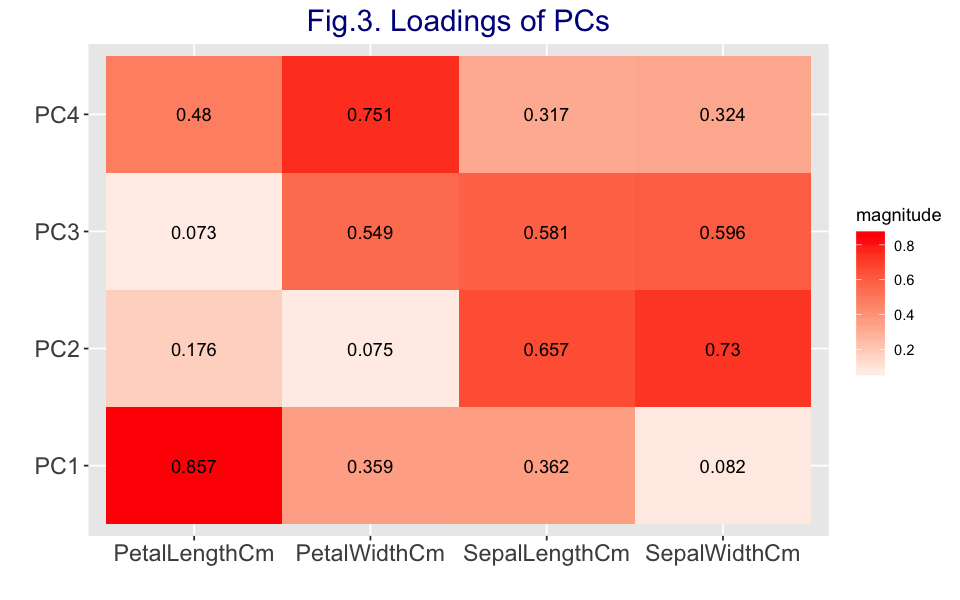#### Principal components (PCs)¶

Sample values from the PCs are shown below to help us compare with the results from Python and Spark.

In :
round(head(pcp$x,10),5)  PC1PC2PC3PC4 -2.68421-0.32661 0.02151 0.00101 -2.71539 0.16956 0.20352 0.09960 -2.88982 0.13735-0.02471 0.01930 -2.74644 0.31112-0.03767-0.07596 -2.72859-0.33392-0.09623-0.06313 -2.27990-0.74778-0.17433-0.02715 -2.82089 0.08210-0.26425-0.05010 -2.62648-0.17041 0.01580-0.04628 -2.88796 0.57080-0.02734-0.02662 -2.67384 0.10669 0.19153-0.05589 As shown below, the PCs are orthogonal to each other (their correlations are zero). In : round(cor(pcp$x), 5)

PC1PC2PC3PC4
PC11000
PC20100
PC30010
PC40001

We can also plot the PCs and color them by the species. Let's take the first two PCs, which account for about 98% of the variance, and see the pattern. We can observe from the figure below that using the first two PCs, we are able to identify two distinct clusters: one for setosa and another one for versicolor and viginica.

In :
df = as.data.frame(pcp$x) df$Species = species
df %>% ggplot(aes(x = PC1, y = PC2, color = Species)) + geom_point() +
ggtitle('Fig.4. The first two PCs') + theme(axis.title = element_text(size=14),
plot.title = element_text(size = 18, hjust = 0.5),
axis.text = element_text(size=14))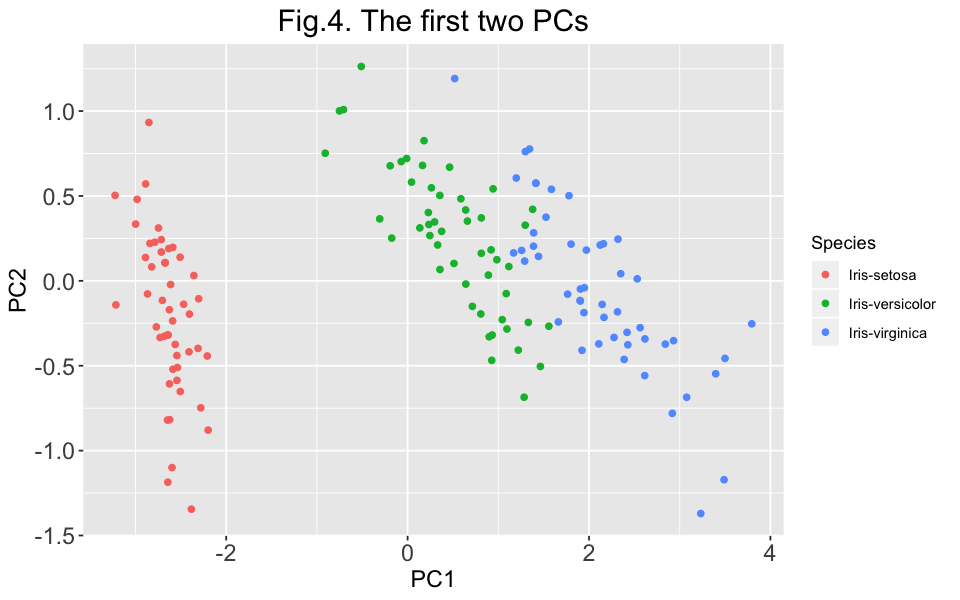#### Let's quickly check the results using SVD¶

In :
scaled_data = scale(iris,center = TRUE, scale = FALSE )
my_svd  = svd(scaled_data)


#### The v matrix from svd is rotation in prcomp¶

In :
all(my_svd$v, pcp$rotation)

TRUE

#### The principal component from prcomp is the product of the original data and matrix v from svd.¶

In :
all(scaled_data %*% my_svd$v, pcp$x)

TRUE

#### For svd(), d = sqrt(ss(fit))¶

In :
all(my_svd$d^2, pcp$x^2 * (nrow(scaled_data) - 1))

TRUE

### Python¶

In Python, unlike R, the PCA decomposition will not center or scale the input data automatically. There is no parameter that controls whether to center or scale the data. We have to process our data before applying PCA.

In :
from sklearn.decomposition import PCA
import pandas as pd
import numpy as np
import seaborn as sns
import matplotlib.pyplot as plt
plt.style.use('ggplot')
from sklearn.preprocessing import scale
%matplotlib inline

In :
df = pd.read_csv("https://raw.githubusercontent.com/venky14/Machine-Learning-with-Iris-Dataset/master/Iris.csv")

Out:
Id SepalLengthCm SepalWidthCm PetalLengthCm PetalWidthCm Species
0 1 5.1 3.5 1.4 0.2 Iris-setosa
1 2 4.9 3.0 1.4 0.2 Iris-setosa
2 3 4.7 3.2 1.3 0.2 Iris-setosa
3 4 4.6 3.1 1.5 0.2 Iris-setosa
4 5 5.0 3.6 1.4 0.2 Iris-setosa
In :
df = df.drop(['Id','Species'], axis = 1)


Just to see how to do it in Python as well, let's create a correlation matrix heatmap. As I said above, strong correlation among variables means the first few PCs can explain most of the variation in the data and PCA could be a right application for such kind of datasets.

In :
corr = df.corr()
plt.figure(figsize = (14, 8))
sns.heatmap(corr, cmap="RdBu",annot = True,
xticklabels=corr.columns.values,
yticklabels=corr.columns.values)
plt.title('Fig.5. Correlation matrix')
plt.xticks(size = 16)
plt.yticks(size = 16)
plt.show()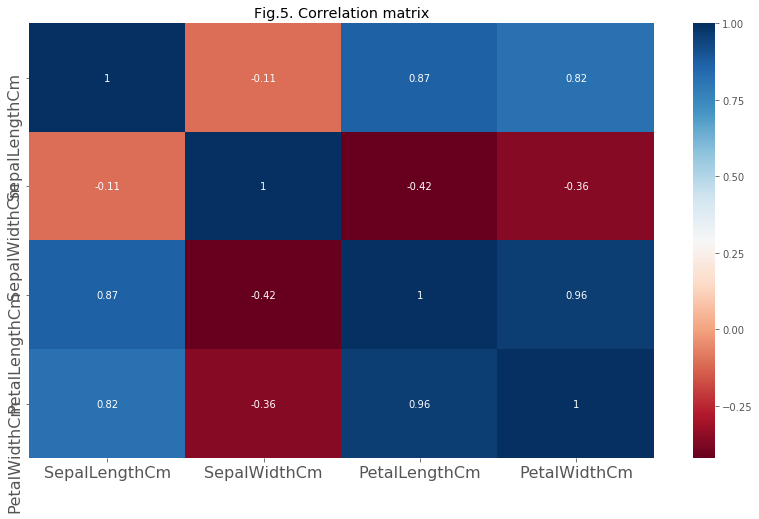Now, let's center the data using the scale function. As you can see below, since we are dealing with variables that have the same unit and similar scales, we do not need to standardize it.

#### Center data¶

In :
scaled = scale(df, with_mean=True, with_std=False)


What does the data look like when the mean of each column is subtracted from each value of each variable?

In :
scaled[:10,:]

Out:
array([[-0.74333333,  0.446     , -2.35866667, -0.99866667],
[-0.94333333, -0.054     , -2.35866667, -0.99866667],
[-1.14333333,  0.146     , -2.45866667, -0.99866667],
[-1.24333333,  0.046     , -2.25866667, -0.99866667],
[-0.84333333,  0.546     , -2.35866667, -0.99866667],
[-0.44333333,  0.846     , -2.05866667, -0.79866667],
[-1.24333333,  0.346     , -2.35866667, -0.89866667],
[-0.84333333,  0.346     , -2.25866667, -0.99866667],
[-1.44333333, -0.154     , -2.35866667, -0.99866667],
[-0.94333333,  0.046     , -2.25866667, -1.09866667]])

Now, let's apply PCA using the centered data.

#### Apply PCA¶

In :
model = PCA()
model.fit(scaled)
transformed = model.transform(scaled)


#### Explained Variance¶

As shown below, the variance explained values we got from R and Python are the same.

In :
np.round(model.explained_variance_ratio_ * 100,4)

Out:
array([92.4616,  5.3016,  1.7185,  0.5183])
In :
var = pd.DataFrame ({'variance':np.round(model.explained_variance_ratio_ * 100,4),
'PC':['p' + str(i) for i in range(1,5)]})

ax = var.plot(kind='bar', figsize=(10,7), color="coral", fontsize=13, legend = False)
ax.set_alpha(0.8)
ax.set_title("Fig. 6. Variance Explained", fontsize=18)
ax.set_xlabel("PC", fontsize=16)
ax.set_ylabel("Variance Explained (%)", fontsize=16)

rects = ax.patches
labels = list(var['variance'])
labels = [str(variance) +'%' for variance in labels]

for rect, label in zip(rects, labels):
height = rect.get_height()
ax.text(rect.get_x() + rect.get_width() / 2, height + 1, label,
ha ='center', va='bottom')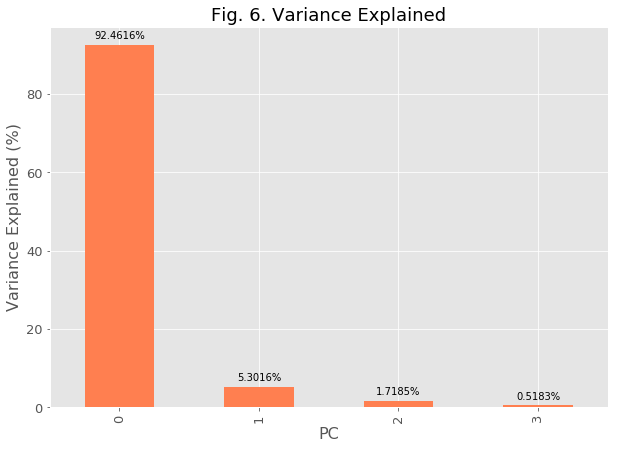The loadings are shown below. The result is an array and as compred to R and Spark, the array is transposed. It is important to note how to access each PCs loading.

In :
loadings = np.round(model.components_,4)

Out:
array([[ 0.3616, -0.0823,  0.8566,  0.3588],
[ 0.6565,  0.7297, -0.1758, -0.0747],
[-0.581 ,  0.5964,  0.0725,  0.5491],
[ 0.3173, -0.3241, -0.4797,  0.7511]])

To access the loading of the first PC, we extract the first row and all the columns as shown below. Note: rows and columns of the loadings have been switched between the results from R and Python.

In :
loadings[0,]

Out:
array([ 0.3616, -0.0823,  0.8566,  0.3588])

#### Principal Components¶

A sample of the principal components are shown below. They are the same with the results from R and Spark. As expected, they are uncorrelated.

In :
np.set_printoptions(suppress=True)
transformed = np.round(transformed, 5)
transformed[:10,:]

Out:
array([[-2.68421,  0.32661, -0.02151,  0.00101],
[-2.71539, -0.16956, -0.20352,  0.0996 ],
[-2.88982, -0.13735,  0.02471,  0.0193 ],
[-2.74644, -0.31112,  0.03767, -0.07596],
[-2.72859,  0.33392,  0.09623, -0.06313],
[-2.2799 ,  0.74778,  0.17433, -0.02715],
[-2.82089, -0.0821 ,  0.26425, -0.0501 ],
[-2.62648,  0.17041, -0.0158 , -0.04628],
[-2.88796, -0.5708 ,  0.02734, -0.02662],
[-2.67384, -0.10669, -0.19153, -0.05589]])
In :
np.round(np.corrcoef(transformed, rowvar=False),5) # If rowvar is True (default), then each row represents a
# variable, with observations in the columns. Otherwise, the relationship
# is transposed: each column represents a variable, while the rows
# contain observations.

Out:
array([[ 1.,  0.,  0., -0.],
[ 0.,  1.,  0.,  0.],
[ 0.,  0.,  1., -0.],
[-0.,  0., -0.,  1.]])

### Spark¶

In :
import findspark
findspark.init()


In Spark, the input data format for PCA is different from that of R and Python. We have to create vector column data using VectorAssembler.

In :
from pyspark import SparkContext
from pyspark.sql import SparkSession
from pyspark.ml.feature import PCA
from pyspark.ml.linalg import Vectors
from pyspark.mllib.regression import LabeledPoint
from pyspark.mllib.util import MLUtils
import numpy as np
from pyspark.ml.feature import StandardScaler
import pyspark.sql.functions as f
import pyspark.sql.types
import pandas as pd
from pyspark.sql import Row
spark = SparkSession.builder.getOrCreate()
sc = SparkContext.getOrCreate()
from pyspark.ml.feature import VectorAssembler

In :
df = pd.read_csv("https://raw.githubusercontent.com/venky14/Machine-Learning-with-Iris-Dataset/master/Iris.csv")
df = df.drop(['Species'], axis = 1)
spark_df = spark.createDataFrame(df)

In :
spark_df.show(10)

+---+-------------+------------+-------------+------------+
| Id|SepalLengthCm|SepalWidthCm|PetalLengthCm|PetalWidthCm|
+---+-------------+------------+-------------+------------+
|  1|          5.1|         3.5|          1.4|         0.2|
|  2|          4.9|         3.0|          1.4|         0.2|
|  3|          4.7|         3.2|          1.3|         0.2|
|  4|          4.6|         3.1|          1.5|         0.2|
|  5|          5.0|         3.6|          1.4|         0.2|
|  6|          5.4|         3.9|          1.7|         0.4|
|  7|          4.6|         3.4|          1.4|         0.3|
|  8|          5.0|         3.4|          1.5|         0.2|
|  9|          4.4|         2.9|          1.4|         0.2|
| 10|          4.9|         3.1|          1.5|         0.1|
+---+-------------+------------+-------------+------------+
only showing top 10 rows



#### Create a single vector column¶

We can use VectorAssembler transformer to combine a given list of columns into a single vector column.

In :
cols = spark_df.drop('Id').columns
cols

Out:
['SepalLengthCm', 'SepalWidthCm', 'PetalLengthCm', 'PetalWidthCm']
In :
assembler = VectorAssembler(inputCols=cols, outputCol = 'features')
output_dat = assembler.transform(spark_df).select('Id', 'features')
output_dat.show(5, truncate = False)

+---+-----------------+
|Id |features         |
+---+-----------------+
|1  |[5.1,3.5,1.4,0.2]|
|2  |[4.9,3.0,1.4,0.2]|
|3  |[4.7,3.2,1.3,0.2]|
|4  |[4.6,3.1,1.5,0.2]|
|5  |[5.0,3.6,1.4,0.2]|
+---+-----------------+
only showing top 5 rows



In Spark, unlike R and like Python, the PCA decomposition will not scale the input data automatically. There is no parameter that controls whether to center or standardize the data. We have to process our data before applying PCA. Now, let's center our data. We do not need to scale it (withStd=False).

#### Center data¶

In :
scaler = StandardScaler(inputCol="features", outputCol="scaledFeatures", withStd=False, withMean=True)

# Compute summary statistics by fitting the StandardScaler
scalerModel = scaler.fit(output_dat)

# Normalize each feature to have unit standard deviation.
scaledData = scalerModel.transform(output_dat)
scaledData.select(['Id', 'scaledFeatures']).show(5, truncate = False) # sample centered data

+---+----------------------------------------------------------------------------------+
|Id |scaledFeatures                                                                    |
+---+----------------------------------------------------------------------------------+
|1  |[-0.7433333333333341,0.44599999999999973,-2.3586666666666667,-0.9986666666666666] |
|2  |[-0.9433333333333334,-0.05400000000000027,-2.3586666666666667,-0.9986666666666666]|
|3  |[-1.1433333333333335,0.1459999999999999,-2.458666666666667,-0.9986666666666666]   |
|4  |[-1.243333333333334,0.04599999999999982,-2.2586666666666666,-0.9986666666666666]  |
|5  |[-0.8433333333333337,0.5459999999999998,-2.3586666666666667,-0.9986666666666666]  |
+---+----------------------------------------------------------------------------------+
only showing top 5 rows



#### Apply PCA¶

In :
pca = PCA(k=4, inputCol = scaler.getOutputCol(), outputCol="pcaFeatures")

model = pca.fit(scaledData)
transformed_feature = model.transform(scaledData)


#### The variance explained are the same with R, Python and Spark¶

In :
np.round(100.00*model.explainedVariance.toArray(),4)

Out:
array([92.4616,  5.3016,  1.7185,  0.5183])

In :
pcs = np.round(model.pc.toArray(),4)
pcs

Out:
array([[-0.3616, -0.6565,  0.581 ,  0.3173],
[ 0.0823, -0.7297, -0.5964, -0.3241],
[-0.8566,  0.1758, -0.0725, -0.4797],
[-0.3588,  0.0747, -0.5491,  0.7511]])

Note: the loadings are in the same structure as in R but in Python they are transposed.

In :
pcs = np.round(model.pc.toArray(),4)
df_pc = pd.DataFrame(pcs, columns = ['PC1','PC2','PC3','PC4'], index = cols)
df_pc

Out:
PC1 PC2 PC3 PC4
SepalLengthCm -0.3616 -0.6565 0.5810 0.3173
SepalWidthCm 0.0823 -0.7297 -0.5964 -0.3241
PetalLengthCm -0.8566 0.1758 -0.0725 -0.4797
PetalWidthCm -0.3588 0.0747 -0.5491 0.7511
In :
df_pc['PC1']

Out:
SepalLengthCm   -0.3616
SepalWidthCm     0.0823
PetalLengthCm   -0.8566
PetalWidthCm    -0.3588
Name: PC1, dtype: float64

The principal components are shown below. They are the same with the results from R and Python. Note: if you run your PCA in Spark, the PCA data may not be in the same order as you get from R and Python from a single machine. This is happening because with Spark in the cluster, the results are collected from different machines and the order does not matter.

In :
transformed_feature.select('pcaFeatures').show(10, truncate = False)

+------------------------------------------------------------------------------------+
|pcaFeatures                                                                         |
+------------------------------------------------------------------------------------+
|[2.6842071251039488,-0.3266073147643876,0.021511837001962353,0.0010061572415422937] |
|[2.7153906156341314,0.16955684755602618,0.20352142500549064,0.09960242401681807]    |
|[2.8898195396179167,0.13734560960502784,-0.024709240998957105,0.019304542832510263] |
|[2.746437197308735,0.3111243157519917,-0.03767197528530131,-0.0759552741085352]     |
|[2.728592981831315,-0.33392456356845435,-0.09622969977460949,-0.0631287327171085]   |
|[2.2798973610095974,-0.7477827132251329,-0.1743256190164031,-0.027146803697900124]  |
|[2.82089068218063,0.08210451102468094,-0.2642510851906963,-0.05009962506284926]     |
|[2.6264819933238193,-0.17040534896028958,0.015801510264314866,-0.046281760966508934]|
|[2.887958565335635,0.5707980263315916,-0.027335406114507355,-0.026615414325655617]  |
|[2.673844686719121,0.10669170375273862,0.19153329973564448,-0.05589096599605492]    |
+------------------------------------------------------------------------------------+
only showing top 10 rows



We can round the PCAs above to compare the results with those from R and Python.

In :
transformed_feature.select('pcaFeatures').rdd.map(lambda x: Row(pcaFeatures = str([x for x in list(np.round(x.\
toArray(),4))]))).toDF().show(truncate = False)

+-----------------------------------+
|pcaFeatures                        |
+-----------------------------------+
|[2.6842, -0.3266, 0.0215, 0.001]   |
|[2.7154, 0.1696, 0.2035, 0.0996]   |
|[2.8898, 0.1373, -0.0247, 0.0193]  |
|[2.7464, 0.3111, -0.0377, -0.076]  |
|[2.7286, -0.3339, -0.0962, -0.0631]|
|[2.2799, -0.7478, -0.1743, -0.0271]|
|[2.8209, 0.0821, -0.2643, -0.0501] |
|[2.6265, -0.1704, 0.0158, -0.0463] |
|[2.888, 0.5708, -0.0273, -0.0266]  |
|[2.6738, 0.1067, 0.1915, -0.0559]  |
|[2.5065, -0.6519, 0.0693, -0.0166] |
|[2.6131, -0.0215, -0.1077, -0.1577]|
|[2.7874, 0.2277, 0.2003, -0.0072]  |
|[3.2252, 0.5033, -0.0684, -0.0219] |
|[2.6435, -1.1862, 0.1445, 0.157]   |
|[2.3839, -1.3448, -0.2837, 0.0019] |
|[2.6225, -0.8181, -0.1453, 0.1647] |
|[2.6483, -0.3191, -0.0334, 0.0761] |
|[2.1991, -0.8792, 0.1145, 0.0253]  |
|[2.5873, -0.5205, -0.2196, -0.0691]|
+-----------------------------------+
only showing top 20 rows



The results so far are the same with all the tools.

## Variables with different units¶

So as mentioned above, when the units are different and the scale varies quite significatly, we have to standardize our data. For this example, let's use climate change data. The data is available here

### R¶

In :
df = read_csv('https://courses.edx.org/asset-v1:[email protected]+block/climate_change.csv')

YearMonthMEICO2CH4N2OCFC-11CFC-12TSIAerosolsTemp
1983 5 2.556 345.96 1638.59 303.677 191.324 350.113 1366.1020.0863 0.109
1983 6 2.167 345.52 1633.71 303.746 192.057 351.848 1366.1210.0794 0.118
1983 7 1.741 344.15 1633.22 303.795 192.818 353.725 1366.2850.0731 0.137
1983 8 1.130 342.25 1631.35 303.839 193.602 355.633 1366.4200.0673 0.176
1983 9 0.428 340.17 1648.40 303.901 194.392 357.465 1366.2340.0619 0.149
1983 10 0.002 340.30 1663.79 303.970 195.171 359.174 1366.0590.0569 0.093

Just as a side note, how has the global temperature been changing in the last 30+ years?

In :
df %>% ggplot(aes(x = Year, y = Temp)) + geom_bar(stat = 'identity') +
ggtitle('Fig. 7. Global Temperature Anomaly') +
theme(axis.title = element_text(size=14), plot.title = element_text(size = 18, hjust = 0.5),
axis.text = element_text(size=14))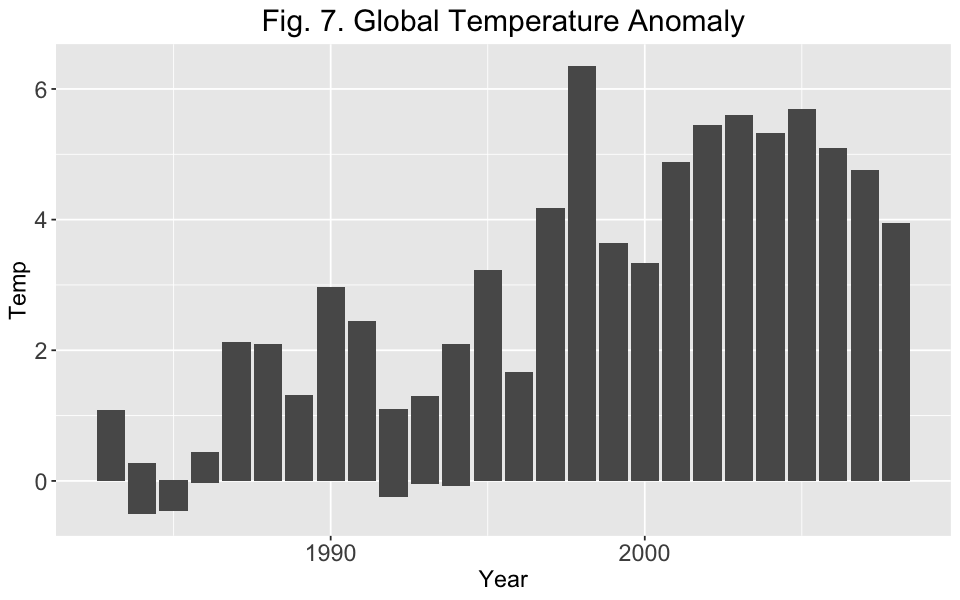If we see the correlation matrix below, we observe that some of the features are strongly correlated. If we want to build a model that predicts global temperature anomaly, rather than using all the columns, it would make sense to first apply PCA and consider only the PCs that explain large portion of the variability in the data (for example 99% of the variance: this depends on the application. In some case 90% or 95% of the variance could be enough.)

#### Correlation matrix¶

In :
correlations = cor(df %>% select(-Temp))
corrplot(correlations, method="number", diag=FALSE,  title = 'Fig.8. Correlation', mar=c(0,0,1,0))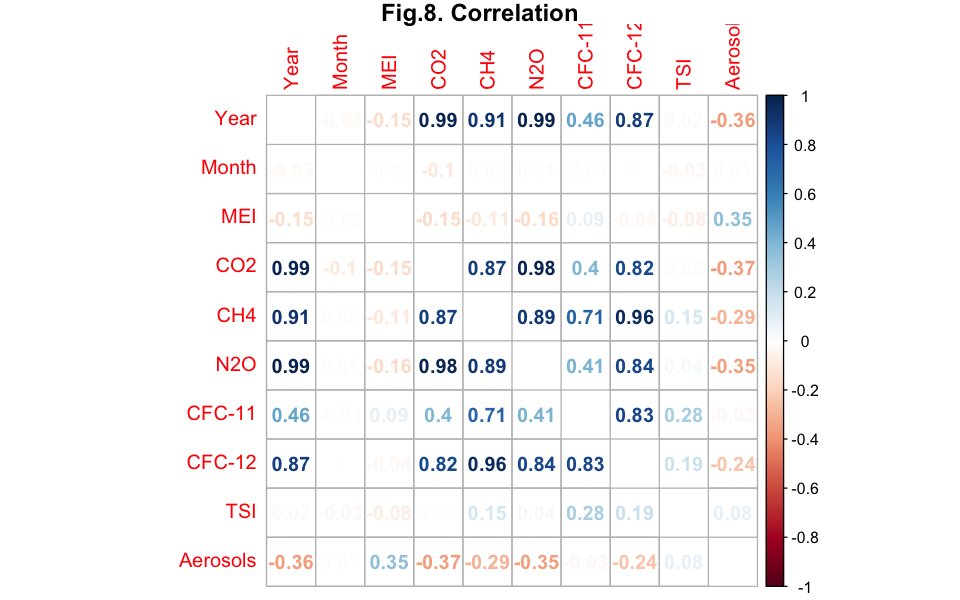As shown below, the last four PCs explain less than 1% of the variance. So we can safely exclude them from our modeling efforts.

#### Apply PCA¶

Now, since the variables have different units, I am also standardizing the data by setting scale. = TRUE

#### The PCs are equal to the V matrix from SVD when the data is centered and scaled.¶

In :
pca1 = prcomp(df %>% select(-Temp), center = TRUE, scale. = TRUE)

In :

scaled = scale(df %>% select(-Temp), center = TRUE, scale = TRUE)
my_svd  = svd(scaled)
all(pca1$x, my_svd$v)

TRUE

#### Variance Explained¶

In :
variance = data_frame(PC = paste0('PC', seq(1,10)), variance = round(100.00*pca1$sdev^2/sum(pca1$sdev^2),4)) %>%
mutate(PC = factor(PC,levels = PC[order(variance,decreasing =F)]))
variance %>% ggplot(aes(x = PC, y = variance)) + geom_bar(stat = 'identity', fill = 'skyblue') + ggtitle('Fig.9. Variance Explained') +
theme(axis.title = element_text(size=14), plot.title = element_text(size = 16,colour="darkblue", hjust = 0.5),
axis.text = element_text(size=14)) + geom_text(aes(label = variance), hjust =0.5) + xlab('') +
ylab('Variance Explained (%)') + coord_flip()